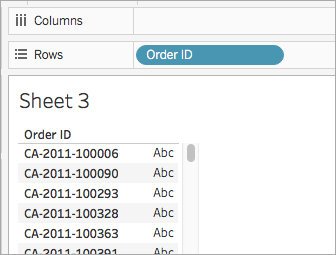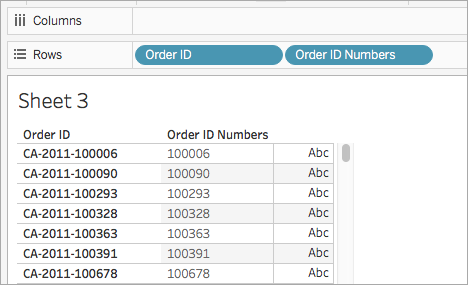# String Functions

This article introduces string functions and their uses in Tableau. It also demonstrates how to create a string calculation using an example.

## Why use string functions

String functions allow you to manipulate string data (i.e. data made of text). Tableau uses the current International Components for Unicode (ICU) library when comparing strings. The way strings are sorted and compared is based both on language and locale, and it’s possible for vizzes to change as the ICU is continuously updated for better language support.

For example, you might have a field that contains all of your customers' first and last names. One member might be: Jane Johnson. You can pull the last names from all your customers into a new field using a string function.

The calculation might look something like this:

`SPLIT([Customer Name], ' ', 2)`

Therefore, `SPLIT('Jane Johnson' , ' ', 2) = `'Johnson'.

## String functions available in Tableau

### ASCII

 Syntax `ASCII(string)` Output Number Definition Returns the ASCII code for the first character of a ``. Example `ASCII('A') = 65` Notes This is the inverse of the `CHAR` function.

### CHAR

 Syntax `CHAR(number)` Output String Definition Returns the character encoded by the ASCII code ``. Example `CHAR(65) = 'A'` Notes This is the inverse of the `ASCII` function.

### CONTAINS

 Syntax `CONTAINS(string, substring)` Output Boolean Definition Returns true if the given string contains the specified substring. Example `CONTAINS("Calculation", "alcu") = true` Notes See also the logical function(Link opens in a new window) `IN` as well as supported RegEx in the additional functions documentation(Link opens in a new window).

### ENDSWITH

 Syntax `ENDSWITH(string, substring)` Output Boolean Definition Returns true if the given string ends with the specified substring. Trailing white spaces are ignored. Example `ENDSWITH("Tableau", "leau") = true` Notes See also the supported RegEx in the additional functions documentation(Link opens in a new window).

### FIND

 Syntax `FIND(string, substring, [start])` Output Number Definition Returns the index position of substring in string, or 0 if the substring isn't found. The first character in the string is position 1.If the optional numeric argument `start` is added, the function ignores any instances of the substring that appear before the starting position. Example `FIND("Calculation", "alcu") = 2``FIND("Calculation", "Computer") = 0``FIND("Calculation", "a", 3) = 7``FIND("Calculation", "a", 2) = 2``FIND("Calculation", "a", 8) = 0` Notes See also the supported RegEx in the additional functions documentation(Link opens in a new window).

### FINDNTH

 Syntax `FINDNTH(string, substring, occurrence)` Output Number Definition Returns the position of the nth occurrence of substring within the specified string, where n is defined by the occurrence argument. Example `FINDNTH("Calculation", "a", 2) = 7` Notes `FINDNTH` is not available for all data sources.See also the supported RegEx in the additional functions documentation(Link opens in a new window).

### LEFT

 Syntax ` LEFT(string, number)` Output String Definition Returns the left-most `` of characters in the string. Example `LEFT("Matador", 4) = "Mata"` Notes See also MID and RIGHT.

### LEN

 Syntax `LEN(string)` Output Number Definition Returns the length of the string. Example `LEN("Matador") = 7` Notes Not to be confused with the spatial function(Link opens in a new window) `LENGTH`.

### LOWER

 Syntax `LOWER(string)` Output String Definition Returns the provided `` in all lowercase characters. Example `LOWER("ProductVersion") = "productversion"` Notes See also UPPER and PROPER.

### LTRIM

 Syntax ` LTRIM(string)` Output String Definition Returns the provided `` with any leading spaces removed. Example `LTRIM(" Matador ") = "Matador "` Notes See also RTRIM.

### MAX

 Syntax `MAX(expression)` or `MAX(expr1, expr2)` Output Same data type as the argument, or `NULL `if any part of the argument is null. Definition Returns the maximum of the two arguments, which must be of the same data type.`MAX` can also be applied to a single field as an aggregation. Example `MAX(4,7) = 7MAX(#3/25/1986#, #2/20/2021#) = #2/20/2021# MAX([Name]) = "Zander"` Notes For strings`MAX` is usually the value that comes last in alphabetical order.For database data sources, the `MAX` string value is highest in the sort sequence defined by the database for that column.For datesFor dates, the `MAX` is the most recent date. If `MAX` is an aggregation, the result will not have a date hierarchy. If `MAX` is a comparison, the result will retain the date hierarchy.As an aggregation`MAX(expression)` is an aggregate function and returns a single aggregated result. This displays as `AGG(expression)` in the viz.As a comparison`MAX(expr1, expr2)` compares the two values and returns a row-level value.See also `MIN`.

### MID

 Syntax `(MID(string, start, [length])` Output String Definition Returns a string starting at the specified `start` position. The first character in the string is position 1.If the optional numeric argument `length` is added, the returned string includes only that number of characters. Example `MID("Calculation", 2) = "alculation"``MID("Calculation", 2, 5) ="alcul"` Notes See also the supported RegEx in the additional functions documentation(Link opens in a new window).

### MIN

 Syntax `MIN(expression)` or `MIN(expr1, expr2)` Output Same data type as the argument, or `NULL `if any part of the argument is null. Definition Returns the maximum of the two arguments, which must be of the same data type.`MIN `can also be applied to a single field as an aggregation. Example `MIN(4,7) = 4MIN(#3/25/1986#, #2/20/2021#) = #3/25/1986#MIN([Name]) = "Abebi"` Notes For strings`MIN` is usually the value that comes first in alphabetical order.For database data sources, the `MIN` string value is lowest in the sort sequence defined by the database for that column.For datesFor dates, the `MIN` is the earliest date. If `MIN` is an aggregation, the result will not have a date hierarchy. If `MIN` is a comparison, the result will retain the date hierarchy.As an aggregation`MIN(expression)` is an aggregate function and returns a single aggregated result. This displays as `AGG(expression)` in the viz.As a comparison`MIN(expr1, expr2)` compares the two values and returns a row-level value.See also `MAX`.

### PROPER

 Syntax `PROPER(string)` Output String Definition Returns the provided `` with the first letter of each word capitalised and the remaining letters in lowercase. Example `PROPER("PRODUCT name") = "Product Name"``PROPER("darcy-mae") = "Darcy-Mae"` Notes Spaces and non-alphanumeric characters such as punctuation are treated as separators.See also LOWER and UPPER.

### REPLACE

 Syntax `REPLACE(string, substring, replacement` Output String Definition Searches `` for `` and replaces it with ``. If `` is not found, the string is not changed. Example `REPLACE("Version 3.8", "3.8", "4x") = "Version 4x"` Notes See also `REGEXP_REPLACE` in the additional functions documentation(Link opens in a new window).
 Syntax `RIGHT(string, number)` Output String Definition Returns the right-most `` of characters in the string. Example `RIGHT("Calculation", 4) = "tion"` Notes See also LEFT and MID.

### RTRIM

 Syntax `RTRIM(string)` Output String Definition Returns the provided `` with any trailing spaces removed. Example `RTRIM(" Calculation ") = " Calculation"` Notes See also LTRIM and TRIM.

### SPACE

 Syntax `SPACE(number)` Output String (specifically, just spaces) Definition Returns a string that is composed of the specified number of repeated spaces. Example `SPACE(2) = " "`

### SPLIT

 Syntax `SPLIT(string, delimiter, token number)` Output String Definition Returns a substring from a string, using a delimiter character to divide the string into a sequence of tokens. Example `SPLIT ("a-b-c-d", "-", 2) = "b"``SPLIT ("a|b|c|d", "|", -2) = "c"` Notes The string is interpreted as an alternating sequence of delimiters and tokens. So for the string `abc-defgh-i-jkl`, where the delimiter character is '`-`', the tokens are (1) `abc`, (2) `defgh`, (3) `i` and (4) `jlk`.`SPLIT` returns the token corresponding to the token number. When the token number is positive, tokens are counted starting from the left end of the string; when the token number is negative, tokens are counted starting from the right.See also supported RegEX in the additional functions documentation(Link opens in a new window). Database limitations The split and custom split commands are available for the following data sources types: Tableau data extracts, Microsoft Excel, Text File, PDF File, Salesforce, OData, Microsoft Azure Market Place, Google Analytics, Vertica, Oracle, MySQL, PostgreSQL, Teradata, Amazon Redshift, Aster Data, Google Big Query, Cloudera Hadoop Hive, Hortonworks Hive and Microsoft SQL Server.Some data sources impose limits on splitting strings. See SPLIT function limitations later in this topic.

### STARTSWITH

 Syntax `STARTSWITH(string, substring)` Output Boolean Definition Returns true if `string` starts with `substring`. Leading white spaces are ignored. Example `STARTSWITH("Matador, "Ma") = TRUE` Notes See also CONTAINS, as well as supported RegEX in the additional functions documentation(Link opens in a new window).

### TRIM

 Syntax `TRIM(string)` Output String Definition Returns the provided `` with leading and trailing spaces removed. Example `TRIM(" Calculation ") = "Calculation"` Notes See also LTRIM and RTRIM.

### UPPER

 Syntax `UPPER(string)` Output String Definition Returns the provided `` in all uppercase characters. Example `UPPER("Calculation") = "CALCULATION"` Notes See also PROPER and LOWER.

## Create a string calculation

Follow along with the steps below to learn how to create a string calculation.

1. In Tableau Desktop, connect to the Sample - Superstore saved data source, which comes with Tableau.

2. Navigate to a worksheet.

3. From the Data pane, under Dimensions, drag Order ID to the Rows shelf.Notice that every order ID contains values for country (CA and US, for example), year (2011) and order number (100006). For this example, you will create a calculation to pull only the order number from the field.

4. Select Analysis > Create Calculated Field.

5. In the calculation editor that opens, do the following:

• Name the calculated field Order ID Numbers.

• Enter the following formula:

`RIGHT([Order ID], 6)`

This formula takes the specified digits (6) from the right of the string and pulls them into a new field.

Therefore, `RIGHT('CA-2011-100006' , 6)` = '100006'.

• When finished, click OK.

The new calculated field appears under Dimensions in the Data pane. Just like your other fields, you can use it in one or more visualisations.

6. From the Data pane, drag Order ID Numbers to the Rows shelf. Place it to the right of Order ID.

Notice how the fields differ now.## SPLIT limitations by data source

Some data sources impose limits on splitting string. The following table shows which data sources support negative token numbers (splitting from the right) and whether there is a limit on the number of splits allow per data source.

A `SPLIT` function that specifies a negative token number and would be legal with other data sources will return the error with these data sources: "Splitting from right is not supported by the data source."

 Data Source Left/Right Constraints Maximum Number of Splits Version limitations Tableau Data Extract Both Infinite Microsoft Excel Both Infinite Text file Both Infinite Salesforce Both Infinite OData Both Infinite Google Analytics Both Infinite Tableau Data Server Both Infinite Supported in version 9.0. Vertica Left only 10 Oracle Left only 10 MySQL Both 10 PostgreSQL Left only prior to version 9.0; both for version 9.0 and above 10 Teradata Left only 10 Version 14 and later Amazon Redshift Left only 10 Aster Database Left only 10 Google BigQuery Left only 10 Hortonworks Hadoop Hive Left only 10 Cloudera Hadoop Left only 10 Impala supported starting in version 2.3.0. Microsoft SQL Server Both 10 2008 and later